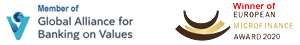##### Thursday November 26, 2020वि.सं २०७७ मङ्सिर ११ बिहिबार# EMI Calculator

### Loan Amount

The value entered must be a number that is greater than or equal to 0 and less than or equal to 50000000.

### Interest Rate

p.a.
The value entered must be a number that is greater than or equal to 8 and less than or equal to 20.

### Loan Term

Years
The value between 1 to 30 Years.
Monthly EMI Rs. 28,752.16

# Simple Interest Calculator

### Loan Amount

The value entered must be a number that is greater than or equal to 0 and less than or equal to 50000000.

### Interest Rate

p.a.
The value entered must be a number that is greater than or equal to 8 and less than or equal to 20.

### Loan Term

Years
The value between 1 to 30 Years.

# Compound Interest Calculator

### Loan Amount

The value entered must be a number that is greater than or equal to 0 and less than or equal to 50000000.

### Interest Rate

p.a.
The value entered must be a number that is greater than or equal to 8 and less than or equal to 20.

### Loan Term

Years
The value between 1 to 30 Years.

# Deposit Calculator

### Deposit Amount

The value entered must be a number that is greater than or equal to 0 and less than or equal to 50000000.

### Interest Rate

p.a.
The value entered must be a number that is greater than or equal to 1 and less than or equal to 10.

### Deposit Term

Years
The value between 1 to 30 Years.